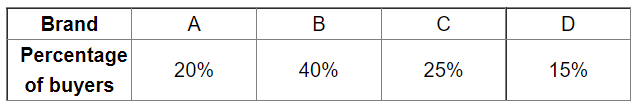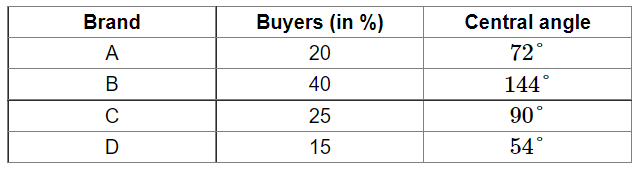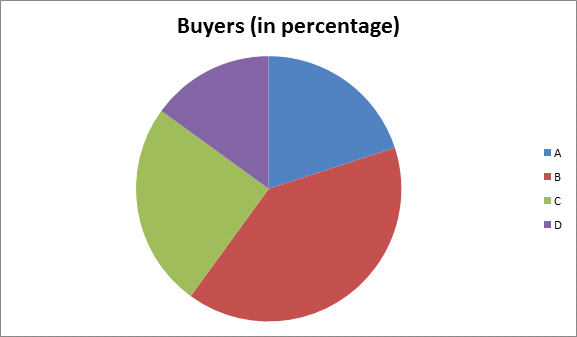# The following table shows the percentages of buyers of four different brands of bathing soaps.

Question:

The following table shows the percentages of buyers of four different brands of bathing soaps.Represent the above data by a pie chart.

Solution:

Total percentage $=100$

Central angle of each brand $=\left(\frac{\text { value (in \%) of each brand }}{100} \times 360\right)^{\circ}$

Calculation of central angles :Construction of pie chart

Steps of construction:

1. Draw a circle of any convenient radius.

2. Draw a horizontal radius of the circle.

3. Draw sectors whose central angles are $72^{\circ}, 144^{\circ}, 90^{\circ}$ and $54^{\circ}$.

4. Shade the sectors so obtained differently and label each one of them.

Thus, we obtain the required pie chart as shown in the figure below.